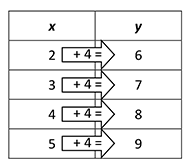Additive Relationship

My Definition

Key Characteristics

The following are true of an additive relationship:

• Two quantities can be expressed as related to each other through addition.

• It can be written as y = x + a, where y is related to x through the addition of a constant, a. The value for a may be positive or negative.

Example

Situation: John will always be four years older than Sue.This table shows an additive relationship between x and y, represented with y = x + 4.

Video Transcript (PDF)

Non-example

Situation: To recreate the recipe, the amount of sugar must always be 12 times the amount of salt. This is a multiplicative relationship. It can be written y = ax, where y is related to x through multiplication by a constant, a.

TEKS: 5(4)(C), 5(4)(D), 6(4)(A), 6(6)(C)